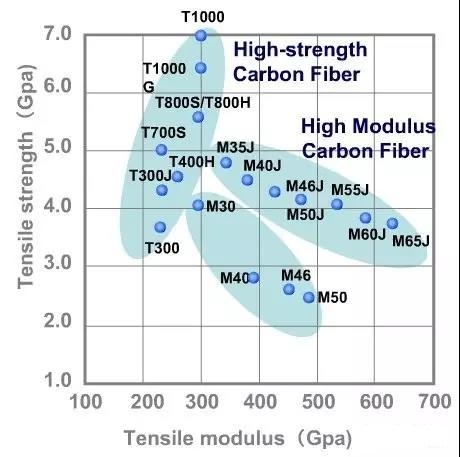# Analysis of the mechanical performance change resulted from the CF materialFigure 1. Carbon Fiber Stress Indicator Chart

Carbon fiber is a kind of inorganic polymer fiber with carbon content of more than 90%, the strength reaches more than 10 times times that of steel.
As shown in the figure, the X-axis is the amount of tensile modulus, and the Y-axis is the tensile strength.
Tensile strength is the maximum tensile force that the material can withstand, and the greater the tensile strength, for example, the maximum tensile strength of the Toray T1100 carbon fiber reaches 6600MPa (millions of Newton per square metre).
The tensile modulus amount refers to the maximum pressure that the material can withstand, and the higher the tensile modulus, the smaller the deformation of the material. The larger the tensile modulus, the greater the tensile strength, but the smaller it may be, so with regard to these materials, we need to combine the actual situation to choose to meet their own needs.

Post time: Mar-25-2019
﻿